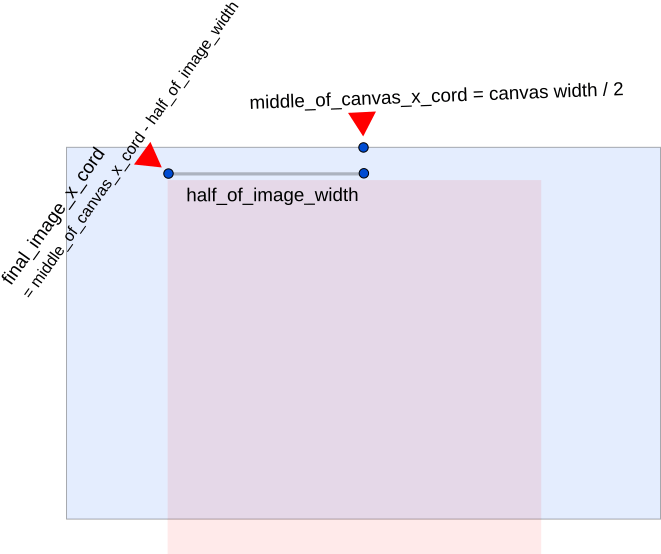# HTML5 :: How To Scale Image To Fit A Canvas (With Demos)

This post has working CodePen demos and code that you can copy paste. I have tried to cover all the situations where you need to scale and image to fit a canvas.

## Situation 1: The Image Is Larger Than The Canvas. It Has To Be Loaded Without Cropping (Scaled Down To FIT)

Here is a sample of what I mean. The image is of size 402×599. We are trying to fit it into a canvas that is 400×400.Here is a working code pen..

See the Pen
Loading An Image Where The Canvas Has A Fixed Size, We FIT The Image Inside The Canvas Without Cropping
on CodePen.

Here is the code where all the magic happens. This is the “call back function” that is called when the image is fully loaded.

```// Setting up a function with the code to run after the image is loaded
// Setting up a function with the code to run after the image is loaded
// Once the image is loaded, we will get the width & height of the image

// get the scale
// it is the min of the 2 ratios
let scale_factor = Math.min(canvas.width/img.width, canvas.height/img.height);

// Lets get the new width and height based on the scale factor
let newWidth = img.width * scale_factor;
let newHeight = img.height * scale_factor;

// get the top left position of the image
// in order to center the image within the canvas
let x = (canvas.width/2) - (newWidth/2);
let y = (canvas.height/2) - (newHeight/2);

// When drawing the image, we have to scale down the image
// width and height in order to fit within the canvas
ctx.drawImage(img, x, y, newWidth, newHeight);
};
```

### How The Code Works (If you are interested)

As you can see on line 10 above, we first try to figure out the “scale factor” needed.

By, “scale factor” I mean, the ratio by which the image needs to be reduced in size.

If we do “400/599” (canvas height / image height) we get: 0.66777963272. This means, the canvas height is 66% lower than the image. Or in other words the image needs to be scaled down by 34% in order to fit into the canvas.

Also if we do, “400/402” (canvas width / image width) we get: 0.99502487562. This means that image image needs to be scaled down by 1% in order to fit into the canvas (height wise).

Now, as you can see above: we have taken the “min” or lower of those 2 numbers as the scale factor. We have chosen to make: 0.66777963272 the scaling down factor. Both the dimensions are going to be made 66% of their original value. With this done, we can be sure that the image will surely fit.

On line 13 & 14 above, calculate the new width and height of the image.

In the next 2 lines: 18 & 19, we figure out where the x & y co-ordinates of the image should be. The formula for this is:

x_cord = middle_of_canvas_x_cord – half_of_image_width

And in order to calculate the “middle_of_canvas_x_cord” we just do: canvas_width / 2

And for the half_of_image we do: (new_image_width) / 2

The following image tries to explain how we get the final x co-ordinate of the image..For finding the “y cord” of the image, we do the same process. But with the canvas and image height.

Finally, we use the “drawImage” function to draw the image based on all the calculations we have done. The MDN docs about the “drawImage” function in case you need to learn more.

## Situation 2: The Image Is Larger Than The Canvas. Cropping is Okay. But, It Needs To FILL The Canvas!

The image is of size 402×599. We are trying to fit it into a canvas that is 400×400. However, this time we need to “fill up the canvas”

Lets see the final result first..The code the does the magic..

```// Setting up a function with the code to run after the image is loaded
// Once the image is loaded, we will get the width & height of the image

// get the scale
// it is the min of the 2 ratios
let scaleFactor = Math.max(canvas.width / img.width, canvas.height / img.height);

// Finding the new width and height based on the scale factor
let newWidth = img.width * scaleFactor;
let newHeight = img.height * scaleFactor;

// get the top left position of the image
// in order to center the image within the canvas
let x = (canvas.width / 2) - (newWidth / 2);
let y = (canvas.height / 2) - (newHeight / 2);

// When drawing the image, we have to scale down the image
// width and height in order to fit within the canvas
ctx.drawImage(img, x, y, newWidth, newHeight);
};```

And the working demo for you to play with..

### How The Code Works (If you are interested)

The code is so similar to the above code, you might not even notice the difference. There is only one 3 letter change.

When calculating the scaling factor, instead of looking for the “min” of the 2 ratios, we look for the “max”. That is all. The rest of the code works exactly the same!